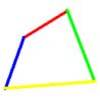# Second EpsilonsThis is our second collection of stage 5 epsilons. An epsilon problem is quick to read and understand. They might not be so easy to solve!

Epsilons will be good for filling spare mathematical moments. Why not carry a few around with you? (Our first collection of epsilons are here).### Baby Circle

##### Age 16 to 18 Challenge Level:

A small circle fits between two touching circles so that all three circles touch each other and have a common tangent? What is the exact radius of the smallest circle?### Upsetting Pitagoras

##### Age 14 to 18 Challenge Level:

Find the smallest integer solution to the equation 1/x^2 + 1/y^2 = 1/z^2### Ab Surd Ity

##### Age 16 to 18 Challenge Level:

Find the value of sqrt(2+sqrt3)-sqrt(2-sqrt3)and then of cuberoot(2+sqrt5)+cuberoot(2-sqrt5).### BT.. Eat Your Heart Out

##### Age 16 to 18 Challenge Level:

If the last four digits of my phone number are placed in front of the remaining three you get one more than twice my number! What is it?### Biggest Bendy

##### Age 16 to 18 Challenge Level:

Four rods are hinged at their ends to form a quadrilateral. How can you maximise its area?### Three Ways

##### Age 16 to 18 Challenge Level:

If x + y = -1 find the largest value of xy by coordinate geometry, by calculus and by algebra.